# range2height

Convert propagated range to target height

Since R2021b

## Syntax

tgtht = range2height(r,anht,el)
tgtht = range2height(r,anht,el,Name=Value)

## Description

example

tgtht = range2height(r,anht,el) returns the target height tgtht as a function of the propagated range r, the sensor height anht, and the local elevation angle el assuming a Curved Earth Model with a 4/3 effective Earth radius.

example

tgtht = range2height(r,anht,el,Name=Value) specifies additional inputs using name-value arguments. For example, you can specify a flat Earth model, a curved Earth model with a given radius, or a CRPL Exponential Reference Atmosphere Model with custom values.

## Examples

collapse all

Determine the target height in meters given a range of 300 km, a sensor height of 10 meters, and an elevation angle of 0.5 degrees. Assume a curved Earth with an effective radius equal to 4/3 times the Earth's actual radius.

R = 300e3; anht = 10; el = 0.5; range2height(R,anht,el)
ans = 7.9325e+03 

Compute target heights in meters using different Earth models and compare the values you obtain. Assume a range of 200 km and an antenna height of 100 meters. Use a range of elevation angles from 0 to 5 degrees.

R = 200e3; anht = 100; el = (0:0.1:5)';

Compute the target height for the given parameters assuming a flat Earth.

tgthtFlat = range2height(R,anht,el,Method="Flat");

Compute the target height for the given parameters assuming free-space propagation with a curved Earth.

r0 = physconst("EarthRadius"); tgthtFS = range2height(R,anht,el,Method="Curved", ... EffectiveEarthRadius=r0);

Compute the target height for the given parameters assuming a 4/3 effective Earth radius.

tgthtEffRad = range2height(R,anht,el);

Compute the target height for the given parameters assuming the CRPL atmospheric model.

tgthtCRPL = range2height(R,anht,el,Method="CRPL");

Plot the results.

plot(el,[tgthtFlat(:) tgthtFS(:) tgthtEffRad(:)], ... el,tgthtCRPL,'--',LineWidth=1.5) grid on xlabel("Elevation Angle (degrees)") ylabel("Target Height (m)") legend(["Flat" "Free Space" "4/3 Earth" "CRPL"],Location="best") title("Target Height Estimation")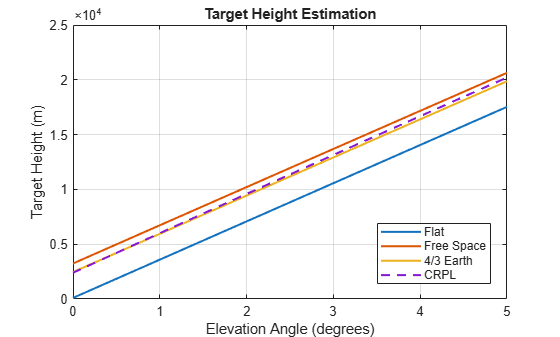## Input Arguments

collapse all

Propagated range between the target and the sensor in meters, specified as a real-valued scalar or vector. If r is a vector, it must have the same size as the other vector input arguments of range2height.

Data Types: double

Sensor height in meters, specified as a nonnegative real-valued scalar or vector. If anht is a vector, it must have the same size as the other vector input arguments of range2height. Heights are referenced to the ground.

Data Types: double

Local elevation angle in degrees, specified as a real-valued scalar or vector. The local elevation angle is the initial elevation angle of the ray leaving the sensor. If el is a vector, it must have the same size as the other vector input arguments of range2height.

Data Types: double

### Name-Value Arguments

Specify optional pairs of arguments as Name1=Value1,...,NameN=ValueN, where Name is the argument name and Value is the corresponding value. Name-value arguments must appear after other arguments, but the order of the pairs does not matter.

Example: Method="CRPL",SurfaceRefractivity=300,RefractionExponent=0.15,MaxNumIterations=8,Tolerance=1e-7

Earth model used for the computation, specified as "Curved", "Flat", or "CPRL".

Data Types: char | string

Effective Earth radius in meters, specified as a positive scalar. If this argument is not specified, range2height calculates the effective Earth radius using a refractivity gradient of –39 × 10–9 N-units/meter, which results in approximately 4/3 of the real Earth radius. This argument applies only if Method is specified as "Curved".

Data Types: double

Surface refractivity in N-units, specified as a nonnegative real-valued scalar. The surface refractivity is a parameter of the CRPL Exponential Reference Atmosphere Model used by range2height. This argument applies only if Method is specified as "CRPL".

Data Types: double

Refraction exponent, specified as a nonnegative real-valued scalar. The refraction exponent is a parameter of the CRPL Exponential Reference Atmosphere Model used by range2height. This argument applies only if Method is specified as "CRPL".

Data Types: double

Maximum number of iterations for the CRPL method, specified as a nonnegative scalar integer. This input acts as a safeguard to preempt long iterative calculations. This argument applies only if Method is specified as "CRPL".

If MaxNumIterations is set to 0, range2height performs a faster but less accurate noniterative CRPL calculation. The noniterative calculation has a maximum height error of 0.056388 m (0.185 ft) at a target height of 30,480 m (100,000 ft) and an elevation angle of 0. The height error for the noniterative method decreases with decreasing target height and increasing elevation angle.

Data Types: double

Numerical tolerance for the CRPL method, specified as a positive real scalar. The iterative process terminates when the numerical tolerance is achieved. This argument applies only if Method is specified as "CRPL" and MaxNumIterations is greater than 0.

Data Types: double

## Output Arguments

collapse all

Target height in meters, returned as a nonnegative real-valued scalar or row vector. If tgtht is a vector, it has the same size as the vector input arguments of range2height. The height is referenced to the ground.

collapse all

### Flat Earth Model

The flat Earth model assumes that the Earth has infinite radius and that the index of refraction of air is uniform throughout the atmosphere. The flat Earth model is applicable over short distances and is used in applications like communications, automotive radar, and synthetic aperture radar (SAR).

Given the antenna height ha and the initial elevation angle θ0, the model relates the target height hT and the slant range RT by

${h}_{T}={h}_{a}+{R}_{T}\mathrm{sin}{\theta }_{0}\text{ }⇔\text{ }{R}_{T}=\left({h}_{T}-{h}_{a}\right)\mathrm{csc}{\theta }_{0},$

so knowing one of those magnitudes enables you to compute the other. The actual range R is equal to the slant range. The true elevation angle θT is equal to the initial elevation angle.

To compute the ground range G, use

$G=\left({h}_{T}-{h}_{a}\right)\mathrm{cot}{\theta }_{0}.$### Curved Earth Model

The fact that the index of refraction of air depends on height can be treated approximately by using an effective Earth's radius larger than the actual value.

Given the effective Earth's radius R0, the antenna height ha, and the initial elevation angle θ0, the model relates the target height hT and the slant range RT by

${\left({R}_{0}+{h}_{T}\right)}^{2}={\left({R}_{0}+{h}_{a}\right)}^{2}+{R}_{T}^{2}+2{R}_{T}\left({R}_{0}+{h}_{a}\right)\mathrm{sin}{\theta }_{0},$

so knowing one of those magnitudes enables you to compute the other. In particular,

${h}_{T}=\sqrt{{\left({R}_{0}+{h}_{a}\right)}^{2}+{R}_{T}^{2}+2{R}_{T}\left({R}_{0}+{h}_{a}\right)\mathrm{sin}{\theta }_{0}}-{R}_{0}.$

The actual range R is equal to the slant range. The true elevation angle θT is equal to the initial elevation angle.

To compute the ground range G, use

$G={R}_{0}\varphi ={R}_{0}\mathrm{arcsin}\frac{{R}_{T}\mathrm{cos}{\theta }_{0}}{{R}_{0}+{h}_{T}}.$A standard propagation model uses an effective Earth's radius that is 4/3 times the actual value. This model has two major limitations:

1. The model implies a value for the index of refraction near the Earth's surface that is valid only for certain areas and at certain times of the year. To mitigate this limitation, use an effective Earth's radius based on the near-surface refractivity value.

2. The model implies a value for the gradient of the index of refraction that is unrealistically low at heights of around 8 km. To partially mitigate this limitation, use an effective Earth's radius based on the platform altitudes.

For more information, see effearthradius.

### CRPL Exponential Reference Atmosphere Model

Atmospheric refraction evidences itself as a deviation in an electromagnetic ray from a straight line due to variation in air density as a function of height. The Central Radio Propagation Laboratory (CRPL) exponential reference atmosphere model treats refraction effects by assuming that the index of refraction n(h) and the refractivity N decay exponentially with height. The model defines

$N=\left(n\left(h\right)-1\right)×{10}^{6}={N}_{\text{s}}{e}^{-{R}_{\text{exp}}h},$

where Ns is the atmospheric refractivity value (in units of 10–6) at the surface of the earth, Rexp is the decay constant, and h is the height above the surface in kilometers. Thus

$n\left(h\right)=1+\left({N}_{\text{s}}\text{\hspace{0.17em}}×{10}^{-6}\right){e}^{-{R}_{\text{exp}}h}.$

The default value of Ns is 313 N-units and can be modified using the SurfaceRefractivity name-value argument in functions that accept it. The default value of Rexp is 0.143859 km–1 and can be modified using the RefractionExponent name-value argument in functions that accept it.

### CRPL Model Geometry

When the refractivity of air is incorporated into the curved Earth model, the ray paths do not follow a straight line but curve downward. (This statement assumes standard atmospheric propagation and nonnegative elevation angles.) The true elevation angle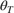is different from the initial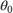. The actual range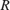, which is the distance along the curved path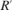, is different from the slant range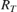.

Given the Earth's radius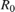, the antenna height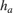, the initial elevation angle, and the height-dependent index of refractionwith value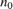at, the modified model relates the target height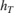and the actual rangebyWhen Method is specified as "CRPL", the integral is solved usingfrom CRPL Exponential Reference Atmosphere Model.

To compute the ground range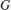, use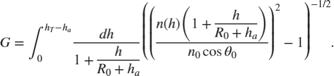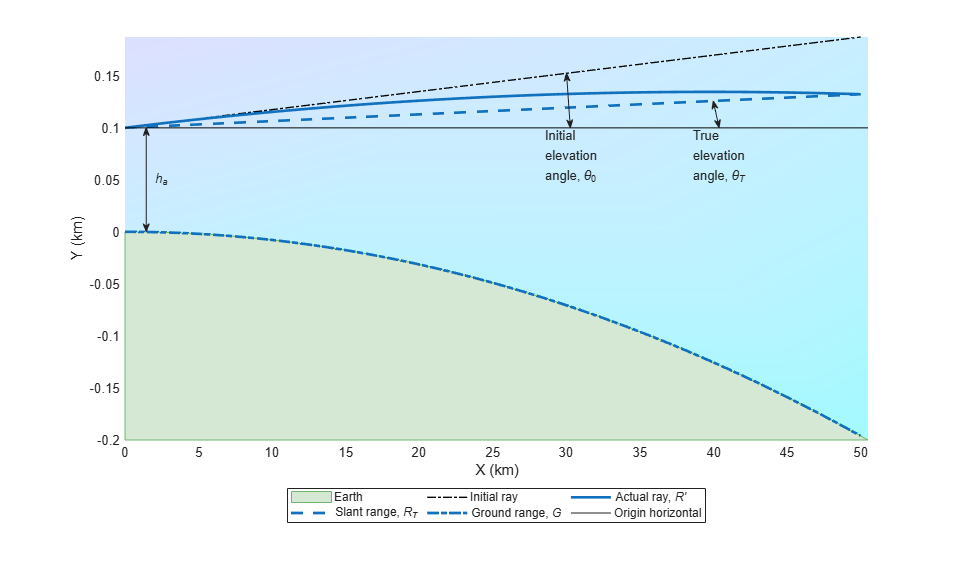Barton, David K. Radar Equations for Modern Radar. Norwood, MA: Artech House, 2013.

 Bean, B.R., and G.D. Thayer. "Central Radio Propagation Laboratory Exponential Reference Atmosphere." Journal of Research of the National Bureau of Standards, Section D: Radio Propagation 63D, no. 3 (November 1959): 315. https://doi.org/10.6028/jres.063D.031.

 Blake, Lamont V. "Ray Height Computation for a Continuous Nonlinear Atmospheric Refractive-Index Profile." Radio Science 3, no. 1 (January 1968): 85–92. https://doi.org/10.1002/rds19683185.